Anúncio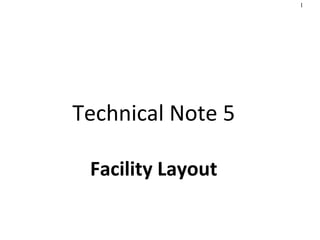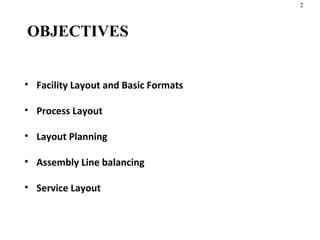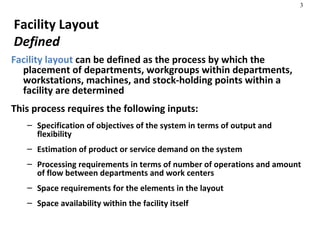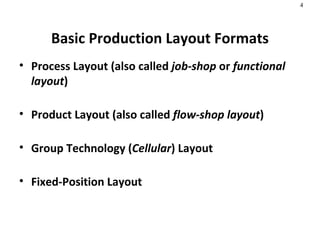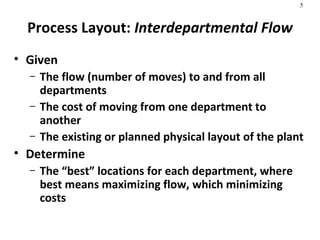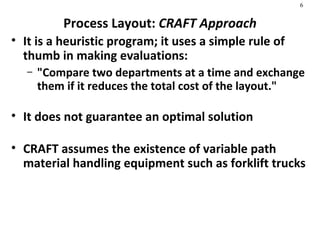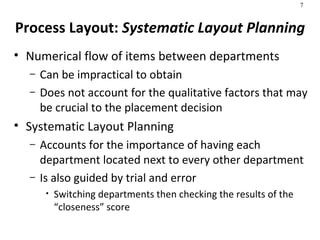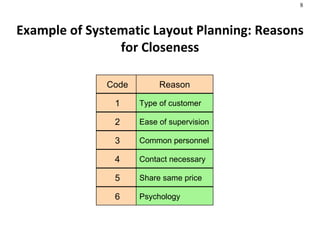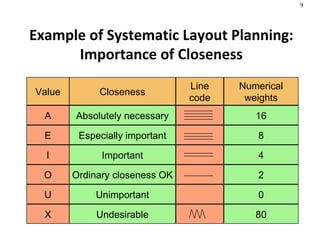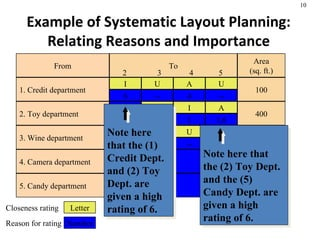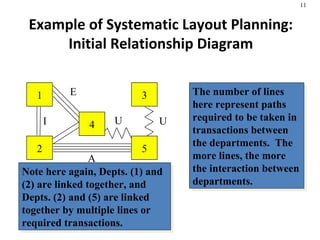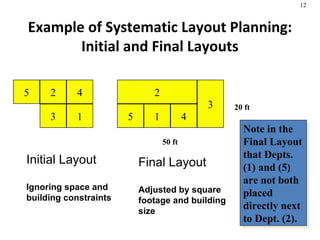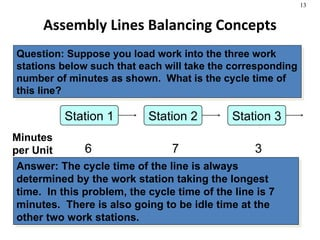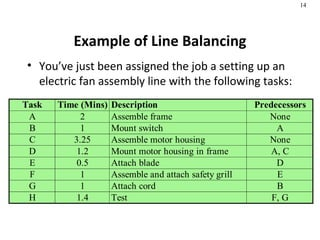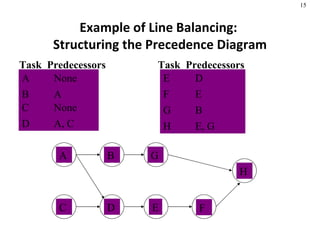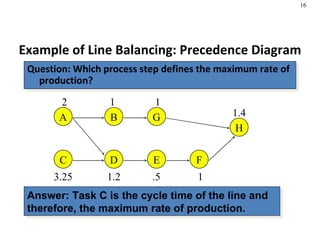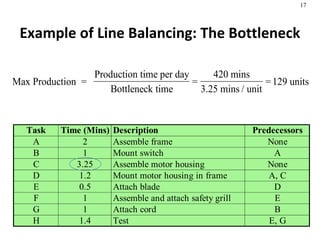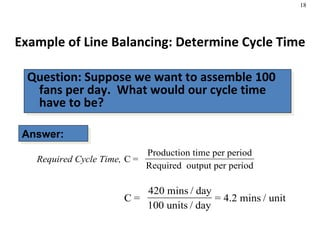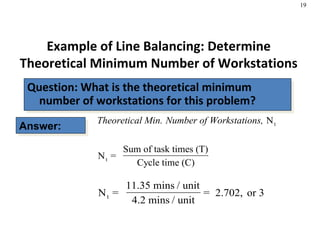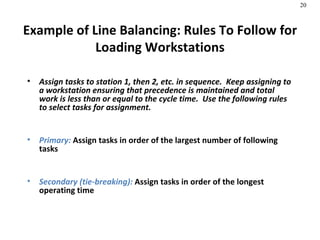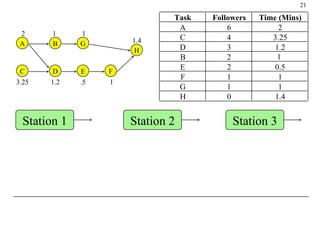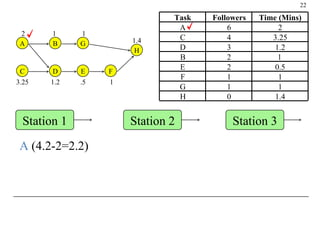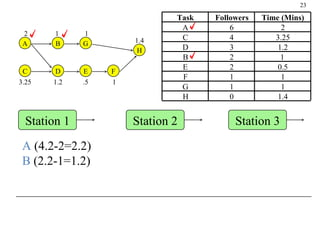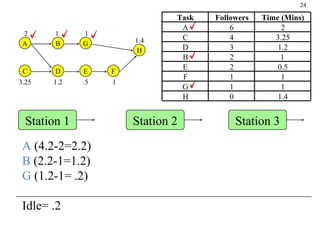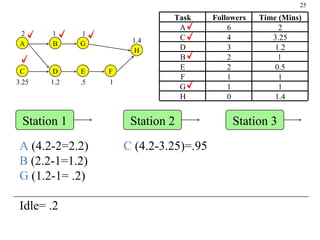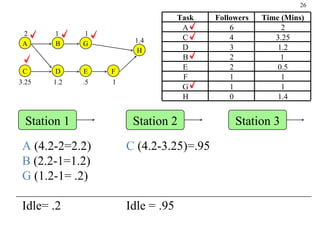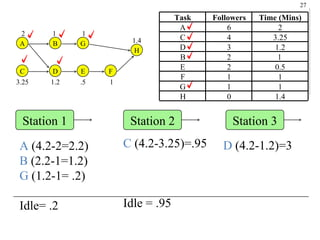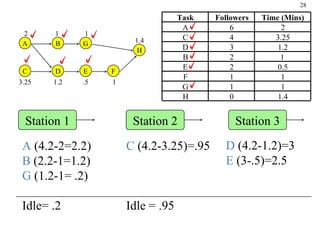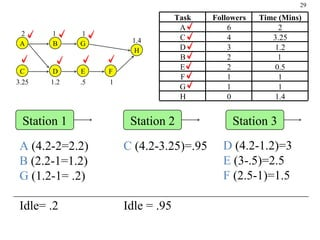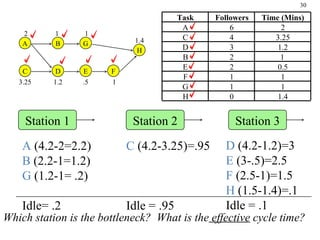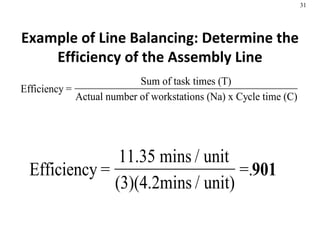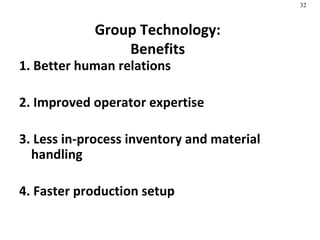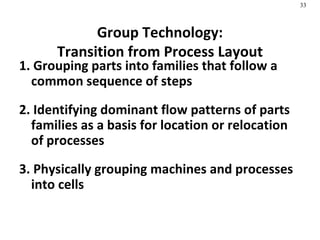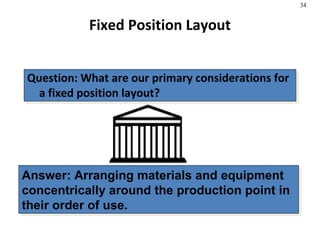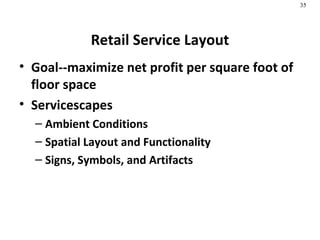1 de 36
Anúncio

### Facility Layout in production management

1. Technical Note 5 Facility Layout
2. Example of Systematic Layout Planning: Reasons for Closeness Code 1 2 3 4 5 6 Reason Type of customer Ease of supervision Common personnel Contact necessary Share same price Psychology
3. Example of Systematic Layout Planning: Importance of Closeness Value A E I O U X Closeness Line code Numerical weights Absolutely necessary Especially important Important Ordinary closeness OK Unimportant Undesirable 16 8 4 2 0 80
4. Example of Systematic Layout Planning: Relating Reasons and Importance From 1. Credit department 2. Toy department 3. Wine department 4. Camera department 5. Candy department -- U 1 I 1,6 A -- U 1 X 1 X To 2 3 4 5 Area (sq. ft.) 100 400 300 100 100 Closeness rating Reason for rating Letter Number 6 I -- U 4 A -- U Note here that the (1) Credit Dept. and (2) Toy Dept. are given a high rating of 6. Note here that the (2) Toy Dept. and the (5) Candy Dept. are given a high rating of 6.
5. Example of Systematic Layout Planning: Initial Relationship Diagram The number of lines here represent paths required to be taken in transactions between the departments. The more lines, the more the interaction between departments. Note here again, Depts. (1) and (2) are linked together, and Depts. (2) and (5) are linked together by multiple lines or required transactions. 1 2 4 3 5 U U E A I
6. Example of Systematic Layout Planning: Initial and Final Layouts Note in the Final Layout that Depts. (1) and (5) are not both placed directly next to Dept. (2). 1 2 4 3 5 Initial Layout Ignoring space and building constraints 2 5 1 4 3 50 ft 20 ft Final Layout Adjusted by square footage and building size
7. Assembly Lines Balancing Concepts Question: Suppose you load work into the three work stations below such that each will take the corresponding number of minutes as shown. What is the cycle time of this line? Answer: The cycle time of the line is always determined by the work station taking the longest time. In this problem, the cycle time of the line is 7 minutes. There is also going to be idle time at the other two work stations. Station 1 Minutes per Unit 6 Station 2 7 Station 3 3
8. Example of Line Balancing: Structuring the Precedence Diagram Task Predecessors A None A B A B C None C D A, C D Task Predecessors E D E F E F G B G H E, G H
9. Example of Line Balancing: The Bottleneck
10. A C B D E F G H 2 3.25 1 1.2 .5 1 1.4 1 Station 1 Station 2 Station 3 Task Followers Time (Mins) A 6 2 C 4 3.25 D 3 1.2 B 2 1 E 2 0.5 F 1 1 G 1 1 H 0 1.4
11. A C B D E F G H 2 3.25 1 1.2 .5 1 1.4 1 Station 1 Station 2 Station 3 A (4.2-2=2.2) Task Followers Time (Mins) A 6 2 C 4 3.25 D 3 1.2 B 2 1 E 2 0.5 F 1 1 G 1 1 H 0 1.4
12. A C B D E F G H 2 3.25 1 1.2 .5 1 1.4 1 A (4.2-2=2.2) B (2.2-1=1.2) Task Followers Time (Mins) A 6 2 C 4 3.25 D 3 1.2 B 2 1 E 2 0.5 F 1 1 G 1 1 H 0 1.4 Station 1 Station 2 Station 3
13. A C B D E F G H 2 3.25 1 1.2 .5 1 1.4 1 A (4.2-2=2.2) B (2.2-1=1.2) G (1.2-1= .2) Idle= .2 Task Followers Time (Mins) A 6 2 C 4 3.25 D 3 1.2 B 2 1 E 2 0.5 F 1 1 G 1 1 H 0 1.4 Station 1 Station 2 Station 3
14. A C B D E F G H 2 3.25 1 1.2 .5 1 1.4 1 C (4.2-3.25)=.95 Task Followers Time (Mins) A 6 2 C 4 3.25 D 3 1.2 B 2 1 E 2 0.5 F 1 1 G 1 1 H 0 1.4 A (4.2-2=2.2) B (2.2-1=1.2) G (1.2-1= .2) Idle= .2 Station 1 Station 2 Station 3
15. C (4.2-3.25)=.95 Idle = .95 A C B D E F G H 2 3.25 1 1.2 .5 1 1.4 1 Task Followers Time (Mins) A 6 2 C 4 3.25 D 3 1.2 B 2 1 E 2 0.5 F 1 1 G 1 1 H 0 1.4 A (4.2-2=2.2) B (2.2-1=1.2) G (1.2-1= .2) Idle= .2 Station 1 Station 2 Station 3
16. C (4.2-3.25)=.95 Idle = .95 A C B D E F G H 2 3.25 1 1.2 .5 1 1.4 1 D (4.2-1.2)=3 Task Followers Time (Mins) A 6 2 C 4 3.25 D 3 1.2 B 2 1 E 2 0.5 F 1 1 G 1 1 H 0 1.4 A (4.2-2=2.2) B (2.2-1=1.2) G (1.2-1= .2) Idle= .2 Station 1 Station 2 Station 3
17. A C B D E F G H 2 3.25 1 1.2 .5 1 1.4 1 C (4.2-3.25)=.95 Idle = .95 D (4.2-1.2)=3 E (3-.5)=2.5 Task Followers Time (Mins) A 6 2 C 4 3.25 D 3 1.2 B 2 1 E 2 0.5 F 1 1 G 1 1 H 0 1.4 A (4.2-2=2.2) B (2.2-1=1.2) G (1.2-1= .2) Idle= .2 Station 1 Station 2 Station 3
18. A C B D E F G H 2 3.25 1 1.2 .5 1 1.4 1 C (4.2-3.25)=.95 Idle = .95 D (4.2-1.2)=3 E (3-.5)=2.5 F (2.5-1)=1.5 Task Followers Time (Mins) A 6 2 C 4 3.25 D 3 1.2 B 2 1 E 2 0.5 F 1 1 G 1 1 H 0 1.4 A (4.2-2=2.2) B (2.2-1=1.2) G (1.2-1= .2) Idle= .2 Station 1 Station 2 Station 3
19. Which station is the bottleneck? What is the effective cycle time? A C B D E F G H 2 3.25 1 1.2 .5 1 1.4 1 C (4.2-3.25)=.95 Idle = .95 D (4.2-1.2)=3 E (3-.5)=2.5 F (2.5-1)=1.5 H (1.5-1.4)=.1 Idle = .1 Task Followers Time (Mins) A 6 2 C 4 3.25 D 3 1.2 B 2 1 E 2 0.5 F 1 1 G 1 1 H 0 1.4 A (4.2-2=2.2) B (2.2-1=1.2) G (1.2-1= .2) Idle= .2 Station 1 Station 2 Station 3
20. Example of Line Balancing: Determine the Efficiency of the Assembly Line
21. End of Technical Note 5

1. 2
2. 2
3. 3
4. 4
5. 6
6. 8
7. 9
8. 10
9. 13
10. 14
11. 15
12. 17
13. 17
14. 18
15. 19
16. 19
17. 21
18. 23
19. 24
20. 25
21. 26
22. 27
23. 28
24. 29
25. 30
26. 31
27. 32
28. 33
29. 34
30. 35
31. 36
32. 37
33. 2
Anúncio